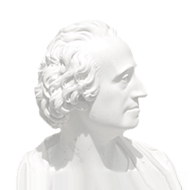• A
• A
• A
• ABC
• ABC
• ABC
• А
• А
• А
• А
• А
Regular version of the site

# Additional Chapters of higher mathematics and statistics

2020/2021
ENG
Instruction in English
4
ECTS credits
Course type:
Elective course
When:
2 year, 3, 4 module

#### InstructorsВецлер Денис Иванович

### Course Syllabus

#### Abstract

Probability Theory and Mathematical Statistics (PT&Stat below) is a core mathematical subject taught to the second year students in the 1st and 2nd academic modules. The material is split between probability theory and statistics almost evenly. The course covers classical probability topics from basic probability to limit theorems. More attention is paid to the conditional moments of multivariate random variables. Depending on the available time more advanced topics such as random walks, the Poisson process and Markov chains may be considered. The statistical section starts with the descriptive techniques but quickly switches to the inferential methods as they are more mathematically involved and require more efforts to explain. The topics covered here include sampling distributions, point and interval estimates, hypothesis testing. We conclude with a univariate and, if time permits, multivariate regression. Throughout the course a certain balance between mathematical rigor and clarity is maintained. Sometimes this dilemma is resolved in favor of illustrative examples which help students capture the main ideas and use them in practice rather than focus on blind memorizing the derivations. However, we find it instructive to provide the tractable proofs whenever it makes pedagogical or some other sense. The course is taught in English and worth 5 credits.#### Learning Objectives

• Knows some of the basic ideas of theoretical statistics, emphasising the applications of these methods and the interpretation of tables and results.
• Is familiar with concepts and methods that provide the foundation for more specialised courses in statistics#### Expected Learning Outcomes

• to be able to use the concepts, terminology, methods and conventions covered in the course to solve mathematical problems from the basic calculus, considered in the course
• to be able to solve unseen mathematical problems involving understanding of the concepts and application of the methods from the course.
• to see how mathematical techniques can be used to solve problems in economics and related subjects.
• apply and be competent users of standard statistical operators and be able to recall a variety of well-known distributions and their respective moments;
• recall a large number of distributions and be a competent user of their mass/density and distribution functions and moment generating functions
• be able to perform inference to test the significance of common measures such as means and proportions and conduct chi-squared tests of contingency tables
• explain the fundamentals of statistical inference and apply these principles to justify the use of an appropriate model and perform hypothesis tests in a number of different settings
• demonstrate understanding that statistical techniques are based on assumptions and the plausibility of such assumptions must be investigated when analysing real problems#### Course Contents

• Basic functions and their applications.
• Differentiation and integration.
• Single-variable optimisation.
• Functions of several variables.
• Constrained optimisation.
• Basics of linear algebra.
• Sequences and series.
• Sampling and statistics.
• Point and interval estimation.
• Hypothesis testing.
• Correlation and linear regression.#### Assessment Elements

• Homeworks
• Quizzes
• Exam of University of London
• Internal HSE Exam#### Interim Assessment

• Interim assessment (4 module)
0.5 * Exam of University of London + 0.25 * Homeworks + 0.2 * Internal HSE Exam + 0.05 * Quizzes#### Recommended Core Bibliography

• Probability and random processes, Grimmett, G. R., Stirzaker, D. R., 2004
• Statistical inference, Casella, G., Berger, R. L., 2002
• Statistical inference, Casella, G., Berger, R. L., 2002
• Statistics for business and economics, Newbold, P., Carlson, W. L., 2010
• Statistics, Freedman, D., Pisani, R., 1998
• Краткий курс высшей математики : учеб. пособие для вузов, Демидович, Б. П., Кудрявцев, В. А., 2001
• Сборник задач и упражнений по математическому анализу : учеб. пособие, Демидович, Б. П., 1997